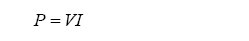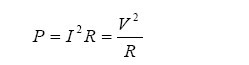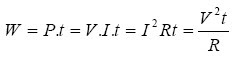## Wednesday, July 22, 2009

### Arus |Tegangan|Daya||Energi Listrik

• Arus listrik|Electric current (I)
Electric current states that the number of load flow in a certain direction on a series of electrical elements of each unit of time. Mathematically expressed by:with,
I: electric current (in ampere)
t: time (in seconds)
Than the electric current magnitude have also have a direction (the direction indicated by arrow), which describes the direction of moving cargo electricity.

Tegangan|Electric current (V)
Electric current Work is needed to move the load of 1 coulomb positive from one point to the tip of the point the other end. Mathematically expressed by:with,
V: voltage (in volt)
W: electrical energy (in Joule)

Usually termed as the voltage potential difference between the two dots on a series of elements. For states marked with a voltage algebra plus-minus polarity (+,-) that point with the other point. As an illustration shown in the picture below:A point means is 5 volt more positive than the point B or point A is -5 volt more negative than point B.

• Daya|Power (P)
Power the rate of electrical energy per unit time to move a load of 1 coulomb through a series of elements. The energy absorbed is proportional to the number of cargo that moved every second (or flow) and comparable with the energy needed to move one coulomb through a series of elements (or tension). Mathematically expressed by:From the above formula can be derived the power formula as follows:Sign agreement to current, voltage, power, and concluded in the following pictureImage shows an edge if the element is V volt more positive towards the end of the other point, and if I enter a flow of elements through the end of the positive, then the power P = VI is absorbed by these elements and vice versa.

• Energy (W)
Energy Is the result of the multiplication with the power of time. Mathematically expressed by:Skema Rangkaian Elektronika